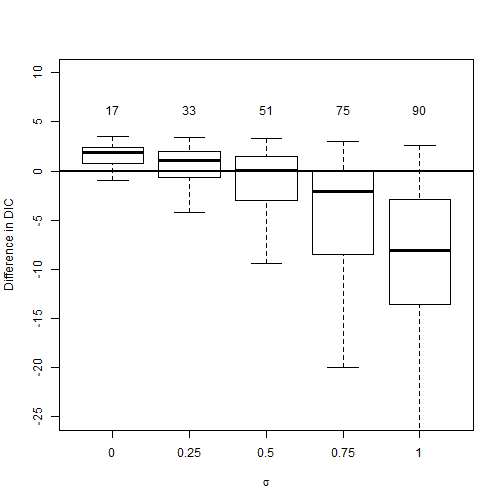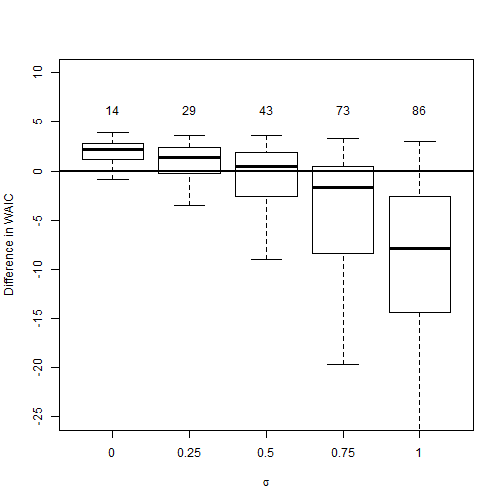# Simulation to compare DIC and WAIC

### Chapter 5.5: Model selection criteria

In the simulation experiment below, we generate data from a known model and evaluate the performance of DIC and WAIC at finding the true data-generating model. The data are simulated to resemble the gambia data in the geoR package. The response $$Y_i$$ is binary and generated from the random effects logistic regression model $\mbox{logit}[\mbox{Prob}(Y_i=1)] = \beta_1 + X_{i}\beta_2 + \theta_{v_i},$ where $$v_i$$ is the village index of observation $$i$$ and $$\theta_v\sim\mbox{Normal}(0,\sigma^2)$$ is the random effect for village $$v$$. Data are generated with $$n=100$$ observations, ten villages each with ten observations, $$\beta_1=0$$, $$\beta_2=1$$ and $$X_i\sim\mbox{Normal}(0,1)$$. We vary the random effect variance $$\sigma^2$$ to determine how large it must be before the model selection criteria consistently favor the random effects model. For each simulated data set we fit two models

1. No random effects: $$\theta_v=0$$

2. Gaussian random effects: $$\theta_v\sim\mbox{Normal}(0,\sigma^2)$$

The priors are $$\beta_1,\beta_2\sim\mbox{Normal}(0,100)$$ and $$\sigma^2\sim\mbox{InvGamma}(0.1,0.1)$$. When data are generated with $$\sigma=0$$ then model 1 is correct, and when $$\sigma>2$$ model 2 is correct. For each model we record the DIC and WAIC for each dataset, and report the number of datasets for which each metric favors the correct model.

## Define the simulation settings

n     <- 100
v     <- rep(1:10,10)
beta1 <- 0
beta2 <- 1
sigma <- c(0.0,0.25,0.5,0.75,1.0)
ns    <- length(sigma)
N     <- 100 # number of simulated datasets

DIC   <- array(0,c(N,2,ns))
dimnames(DIC)[] <- paste("Dataset",1:N)
dimnames(DIC)[] <- c("No RE","RE")
dimnames(DIC)[] <- paste("Sigma =",sigma)

WAIC  <- DIC


## Prep for JAGS

   library(rjags)
burn   <- 1000
iters  <- 11000
chains <- 2

# Define the simple logistic regression model
mod1 <- "model{
for(i in 1:n){
Y[i]          ~ dbern(pi[i])
logit(pi[i]) <- beta+ X[i]*beta
like[i]      <- dbin(Y[i],pi[i],1) # For WAIC computation
}
for(j in 1:2){beta[j] ~ dnorm(0,0.01)}
}"

# Define the random effecs logistic regression model
mod2 <- "model{
for(i in 1:n){
Y[i]          ~ dbern(pi[i])
logit(pi[i]) <- beta + X[i]*beta + theta[v[i]]
like[i]      <- dbin(Y[i],pi[i],1) # For WAIC computation
}
for(j in 1:2){beta[j] ~ dnorm(0,0.01)}
for(j in 1:10){theta[j] ~ dnorm(0,tau)}
tau   ~ dgamma(0.1,0.1)
}"


## Run the simulation

 for(s in 1:ns){for(sim in 1:N){

# Generate data
set.seed(2*0820+sim)

X     <- rnorm(n)
theta <- rnorm(max(v),0,sigma[s])
prob  <- 1/(1+exp(-beta1-beta2*X-theta[v]))
Y     <- rbinom(n,1,prob)

# Model 1 - No random effects

mod    <- textConnection(mod1)
data   <- list(Y=Y,X=X,n=n)
model  <- jags.model(mod,data = data, n.chains=chains,quiet=TRUE)
update(model, burn, progress.bar="none")
samps  <- coda.samples(model, variable.names=c("like"),
n.iter=iters, progress.bar="none")

# Compute DIC
dic           <- dic.samples(model,n.iter=iters,progress.bar="none")
DIC[sim,1,s]  <- sum(dic$dev)+sum(dic$pen)

# Compute WAIC
like          <- rbind(samps[],samps[]) # Combine samples from the two chains
fbar          <- colMeans(like)
Pw            <- sum(apply(log(like),2,var))
WAIC[sim,1,s] <- -2*sum(log(fbar))+2*Pw

# Model 2: Random effects model

mod    <- textConnection(mod2)
data   <- list(Y=Y,X=X,n=n,v=v)
model  <- jags.model(mod,data = data, n.chains=chains,quiet=TRUE)
update(model, burn, progress.bar="none")
samps  <- coda.samples(model, variable.names=c("like"),
n.iter=iters, progress.bar="none")

# Compute DIC
dic           <- dic.samples(model,n.iter=iters,progress.bar="none")
DIC[sim,2,s]  <- sum(dic$dev)+sum(dic$pen)

# Compute WAIC
like          <- rbind(samps[],samps[])
fbar          <- colMeans(like)
Pw            <- sum(apply(log(like),2,var))
WAIC[sim,2,s] <- -2*sum(log(fbar))+2*Pw

}}


## Compile the results

 DIC_diff     <- DIC[,2,]-DIC[,1,]
DIC_pick_RE  <- colMeans(DIC_diff<0,na.rm=TRUE)

WAIC_diff    <- WAIC[,2,]-WAIC[,1,]
WAIC_pick_RE <- colMeans(WAIC_diff<0,na.rm=TRUE)

SIG          <- matrix(sigma,N,ns,byrow=TRUE)
boxplot(DIC_diff~SIG,ylim=c(-25,10),outline=FALSE,
xlab=expression(sigma),ylab="Difference in DIC")
abline(0,0,lwd=2)
text(1:ns,rep(5,ns),round(100*DIC_pick_RE),pos=3)boxplot(WAIC_diff~SIG,ylim=c(-25,10),outline=FALSE,
xlab=expression(sigma),ylab="Difference in WAIC")
abline(0,0,lwd=2)
text(1:ns,rep(5,ns),round(100*WAIC_pick_RE),pos=3)Summary: Both $$WAIC$$ and $$DIC$$ reliably select the correct model. When data are generated with $$\sigma=0$$ the model without random effects is true and the random effects models is only selected for 10-20% of the simulated datasets. On the other hand, when data are generated with $$\sigma>0$$ both metrics select the random effects model with high probability.